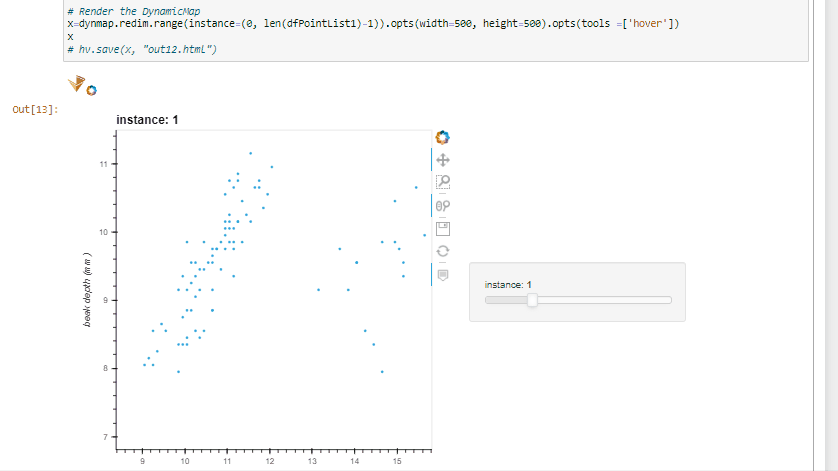# The slider is not working properly for list of holoview points rendered through hv.DynamicMap, skipping some indexes of list when saved as html

``````import holoviews as hv
from holoviews import opts
hv.extension('bokeh')
import panel as pn
import numpy as np
import holoviews as hv
from bokeh.embed import file_html

import panel as pn
import holoviews as hv
import pandas as pd

pn.extension()  # add this line to explicitly load the necessary JavaScript libraries

df_2012 = df.loc[df['year']==2012, :].copy()
df_1973 = df.loc[df['year']==1973, :].copy()
df_1987 = df.loc[df['year']==1987, :].copy()
df_1991 = df.loc[df['year']==1991, :].copy()
df_1975 = df.loc[df['year']==1975, :].copy()

print(np.unique(df['year']))
point1= hv.Points(
data=df_2012,
kdims=['beak length (mm)', 'beak depth (mm)'])

point2 = hv.Points(
data=df_1973,
kdims=['beak length (mm)', 'beak depth (mm)'])

point3 = hv.Points(
data=df_1987,
kdims=['beak length (mm)', 'beak depth (mm)'])

point4 = hv.Points(
data=df_1991,
kdims=['beak length (mm)', 'beak depth (mm)'])

point5 = hv.Points(
data=df_1975,
kdims=['beak length (mm)', 'beak depth (mm)'])

dfPointList1 = [point1, point2, point3, point4, point5]

# Create a list of Holoview points
# Define a function that takes a time parameter and returns the corresponding Holoview point
def plot_points(instance):
index = int(instance) % len(dfPointList1)
return dfPointList1[index]

# Create a DynamicMap object from the plot_points function
dynmap = hv.DynamicMap(plot_points , kdims=['instance'])

# Render the DynamicMap
x=dynmap.redim.range(instance=(0, 5)).opts(width=500, height=500)
x
#hv.save(x, "out12.html")
``````

In the above example I have list of holoview points say dfPointList1, when I am trying to render in a dynamic map it’s working expected in jupyter output. like all the points in the list are being rendered while slide over the instance slider but when I saved it to a html file, some instances are skipped(1,3) indexes of dfPointList1 only {0,2,4} indexes of dfPointList1 got rendered.

Jupyter Notebook Output :All the indexes from the list are being rendered , in slider it’s showing from 0 to 5 as dfPointList size is 5

HTML page Output :Here you can notice some of the indexes are being skipped on the slider. only 0,2,4 indexes of the dfPointList1 are being rendered. index and 1,3 points are skipped in slider.

Would any one suggest what is the issue with above code and is there any solution to same ? You can run the above code in note book to reproduce the issue.